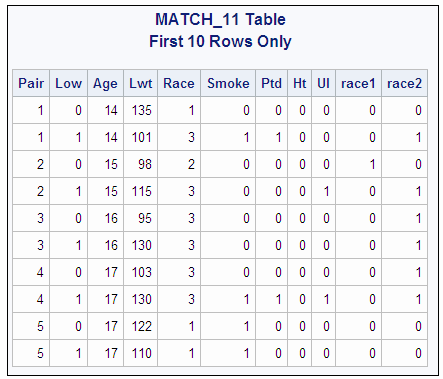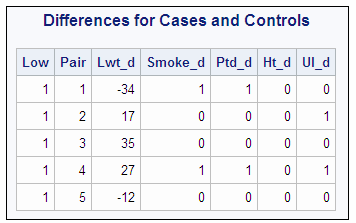# Example 14: Matching Case Rows and Control Rows

 Features: joined-table component Table names: MATCH_11MATCH
This example uses a table that contains data for a case-control study. Each row contains information for a case or a control. To perform statistical analysis, you need a table with one row for each case-control pair. PROC SQL joins the table with itself in order to match the cases with their appropriate controls. After the rows are matched, differencing can be performed on the appropriate columns.
The input table Appendix 3, “MATCH_11,” in Base SAS Procedures Guide contains one row for each case and one row for each control. Pair contains a number that associates the case with its control. Low is 0 for the controls and 1 for the cases. The remaining columns contain information about the cases and controls.
```options ls=120 nodate pageno=1;
proc sql outobs=10;
title 'MATCH_11 Table';
title2 'First 10 Rows Only';
select * from match_11;```
MATCH_11 Table, First 10 Rows## Program

```proc sql;
create table match as
select
one.Low,
one.Pair,
(one.lwt - two.lwt) as Lwt_d,
(one.smoke - two.smoke) as Smoke_d,
(one.ptd - two.ptd) as Ptd_d,
(one.ht - two.ht) as Ht_d,
(one.ui - two.ui) as UI_d```
```from match_11 one, match_11 two
where (one.pair=two.pair and one.low>two.low);```
`   title 'Differences for Cases and Controls';`
```select *
from match(obs=5);```

## Program Description

Create the MATCH table.The SELECT clause specifies the columns for the table MATCH. SQL expressions in the SELECT clause calculate the differences for the appropriate columns and create new columns.
```proc sql;
create table match as
select
one.Low,
one.Pair,
(one.lwt - two.lwt) as Lwt_d,
(one.smoke - two.smoke) as Smoke_d,
(one.ptd - two.ptd) as Ptd_d,
(one.ht - two.ht) as Ht_d,
(one.ui - two.ui) as UI_d```
Specify the type of join and the join criterion.The FROM clause lists the table MATCH_11 twice. Thus, the table is joined with itself. The WHERE clause returns only the rows for each pair that show the difference when the values for control are subtracted from the values for case.
```from match_11 one, match_11 two
where (one.pair=two.pair and one.low>two.low);```
Specify the title.
`   title 'Differences for Cases and Controls';`
Display the first five rows of the MATCH table.The SELECT clause selects all the columns from MATCH. The OBS= data set option limits the printing of the output to five rows.
```select *
from match(obs=5);```

## Output

Differences for Cases and Controls